Updated by Dhansu Series on Aug 30, 2015
REPORTDhansu Series
Owner
14 items   1 followers   0 votes   20 views

# Operational Amplifiers Types Tutorials Series

Operational Amplifiers Types Tutorials Series

1

## Frequency Response of an Opamp - Operational Amplifiers Types Tutorials Series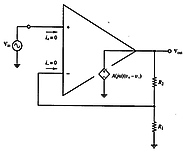An open-loop op-amp has a constant gain Ao only at low frequencies, and a continuously reducing gain at higher frequencies due to internal device and circuit inherent limits.

2

## Slew Rate Limitation in an OpAmp - Operational Amplifiers Types Tutorials Series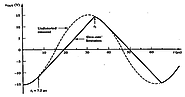Slew Rate Limitation in an Op-amp - Operational Amplifiers Types Tutorials Series.A real op-amp is limited in its ability to respond instantaneously to an input signal with a high rate of change of its input voltage. This limitation is called the slew rate, referring to the maximum rate at which the output can be "slewed".

3

## Non-zero Input Bias Current - Operational Amplifiers Types Tutorials Series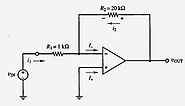Effect of Non-zero Input Bias Currents *In practice op-amps do not actually have zero input currents, but rather have very small input currents labeled I+ and I- in the figure at the left -Modeled as internal current sources inside op-amp -I+ and I- are both the same polarity *e.g.

4

## Output-Offset Voltage and Input Offset Current - Operational Amplifiers Types Tutorials Series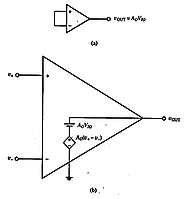Output-Offset Voltage and Nulling Out Offset:-How can we correct for offset voltage? *A parameter called the output-offset voltage may be used to represent the internal imbalance of an op-amp, rather than the input-offset voltage.Consequently, the output-offset voltage is essentially the input-offset voltage multiplied by the open loop gain.

5

## Non- Ideal Properties of OpAmps -I -Operational Amplifiers Types Tutorials Series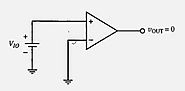Non-Ideal Properties of Op-amps:Input-Offset Voltage (Above Fig):- Output Saturation Voltage:-*We have been assuming v+ = v- when vOUT = 0. In actual practice, however, there is usually a small input (or output) dc offset voltage in order to force vOUT to 0, under open-loop operation.

6

## Schmitt Trigger -Open Loop OpAmps - Operational Amplifiers Types Tutorials Series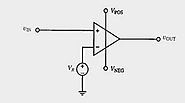Non-Linear Op-amp Circuits-Such non-linear op-amp uses are often found in signal processing applications *Two examples of such non-linear operation are shown at the left-In below fig is an open-loop polarity indicator *Op-amps are sometimes used in non-linear open-loop configurations where the slightest change in vIN will force the op-amp into

7

## OpAmp as a Differentiator - Operational Amplifiers Types Tutorials Series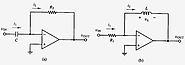Op-amp as a Differentiator *The two op-amp configurations shown below perform the function of differentiation -The circuit on the left is the complement of the integrator circuit shown on slide 2-14, simply switching the capacitor and resistor -The circuit on the right differentiates by replacing the capacitor with an inductor

8

## Op-amp Integrator Network-Operational Amplifiers Types Tutorials Series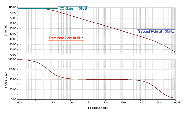1. Shown below is an op-amp integrator network -The output will be equal to the integral of the input, as long as the op-amp remains in its linear region -Due to the virtual short property of the op-amp input, we can write i1 = vIN/R1 -This current i1 starts charging the capacitor C according to the relation i1 = C(dvC/dt).
9

## Op-amp with T-bridge Feedback Network-Operational Amplifiers Types Tutorials Series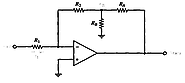Op-amp,T-bridge,Feedback Network,Operational Amplifiers Types,Tutorials Series-Op-amp with T-bridge Feedback Network-To build an op-amp with high closed-loop gain may require a high value resistor R2 which may not be easily obtained in integrated circuits due to its large size.A compromise to eliminate the high value resistor is the op-amp with T-bridge feedback network, shown below :-

10

## Instrumentation and Summation Configuration-Operational Amplifiers Types Tutorials Series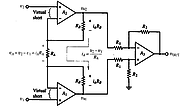Instrumentation Amplifier Configuration Some applications, such as an oscilloscope input, require differential amplification with extremely high input resistance(Circuit is shown above) A1 and A2 are additional op-amps with extremely high input resistances at v1 and v2 (input currents = 0)*Differential gain of input section: -Due to the virtual shorts at

11

## Voltage Follower and Difference Amplifier Configuration-Operational Amplifiers Types Tutorials Series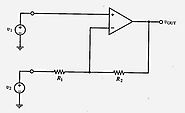1. The op-amp configuration shown above is a voltage-follower often used as a buffer amplifier 2. A typical voltage-follower transfer curve is shown below for the case VPOS = +15V and VNEG = -10V -For vIN between -10 and +15 volts, vOUT = vIN -If vIN exceeds +15V, the output saturates at VPOS -If vIN < -10V, the output saturates at VNEG 3.
12

## Linear Op-amp Operation-Inverting And Non-Inverting Configuration - Operational Amplifiers Tutorials Series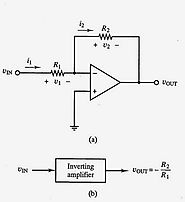An op-amp can use negative feedback to set the closed-loop gain as a function of the circuit external elements (resistors), independent of the op-amp gain, as long as the internal op-amp gain is very high *Shown at left is an ideal op-amp in a non-inverting configuration with negative feedback provided

13

## Operational Amplifiers Tutorials Series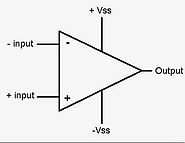Operational Amplifiers circuit symbol is shown abovewhere:V+: non-inverting input V−: inverting input Vout: output VS+: positive power supply VS−: negative power supply Operation The amplifier's differential inputs consist of a non-inverting input (+) with voltage V+ and an inverting input (-) with voltage V−; ideally the op-amp amplifies only the difference in voltage between the two, which is called the differential input voltage.

14

## Input Differential Amplifier - Basics Tutorial 741 IC - Op-Amp Structure And Testing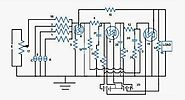An amplifier is a circuit which can produce an output voltage, which is the product of input voltage with a value called voltage gain. An op-amp (operational amplifier) is a kind of amplifier circuit which can perform an operation (addition, subtraction etc.) on the input voltages, apart from simply amplifying the input.